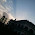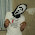### CLASS XI HELP DESK FOR SEPTEMBER TEST

Here students of GMACPS can ask their doubts about September 2012 Exam.

Please allow us 2-3 hours to reply back your queries.

Thank you.
And Best of luck!

September 2012 Test Syllabus (quadd.co.cc)

THIS HELP-DESK IS CLOSED w.e.f. September 19, 2012 6:00 A.M.

1.Ques: Evaluate the following c++ expression where a=5,b=3 and both a and b are of integer data type.

c=a-(b++)*(--a)

Answer is -8 but not getting how according to me it should be -7 but it is -8

2.question.----what is importance of 'stored program concept'?

1.@Nirvair Singh
This concept was introduced by John Von Neumann and the basic idea behind this concept is that a sequence of instructions can be stored in the memory of the computer for automatically directing the flow of operation and this concept enabled computers to be much more powerful and flexible. We can even store data on computer memory and can modify it whenever we want, we can do all this because of this 'stored program concept'.
This concept was first introduced in EDVAC (Electronic Discrete Variable Automatic Computer)

2.ok thanks supreet..

3.Types of assignment operators?

4.Types of assignment operators?

1.Assignment operator (=)
and its shorthand variants ( += *= etc.)

5.on quadd the syllabus is given but max marks given are 80 and at last note is written that q.paper will be of 70 marks......please tell me how much marks will q.paper contain....

1.it will be of 70 marks.
30 marks practical
total = 100

6.what are multi line comments

1.A comment is text that the compiler ignores but is useful for programmers. Comments are normally used to annotate i.e. add explanation etc about the code for future reference.
In C++ multi line comments are written in the following way :
The /* (slash, asterisk) characters, followed by any sequence of characters (including new lines), followed by the */ characters.

2.thanks supreet.....

7.how to change binary to decimal and decimal to binary?........actually i have lost my notebook and now requesting for answer..

1.to change binary to decimal use that multiplication method and for changing decimal to binary use division method.
i hope you are clear about these methods.